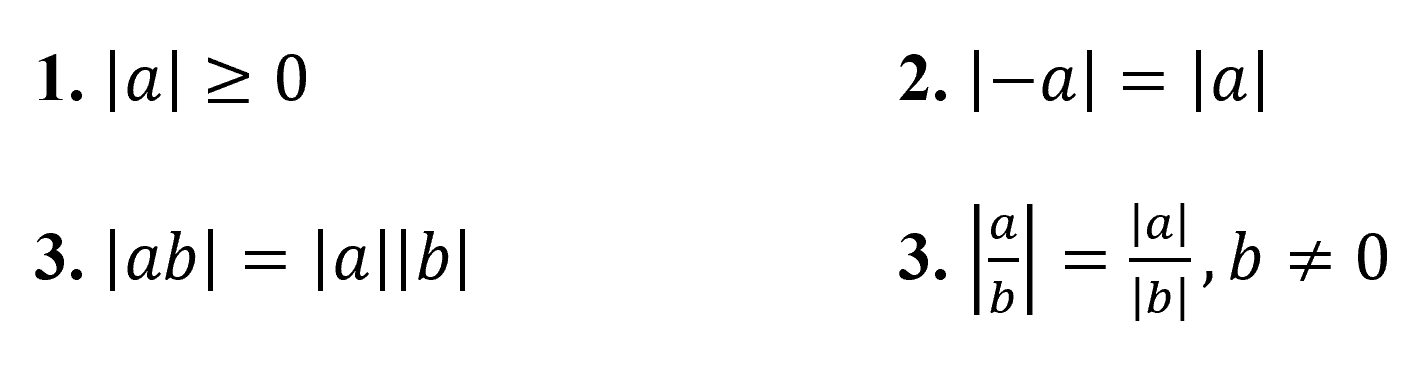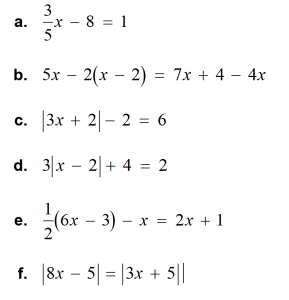MrFerriero@gmail.com

# Solving Linear Equations

Goals

• Solve linear equations using addition and subtraction.
• Solve linear equations using multiplication and division.
• Use linear equations to solve real-life problems.
• Solve multi-step linear equations using inverse operations.
• Use multi-step linear equations to solve real-life problems.
• Use unit analysis to model real-life problems.
• Solve linear equations that have variables on both sides.
• Identify special solutions of linear equations.
• Solve absolute value equations.
• Solve equations involving two absolute values.
• Identify special solutions of absolute value equations.
• Rewrite literal equations.
• Rewrite and use formulas for area.
• Rewrite and use other common formulas.

Vocabulary

• A conjecture is an unproven statement about a general mathematical concept.
• A proven statement about a general mathematical concept is a rule or a theorem.
• An equation is a statement that two expressions are equal.
• A linear equation in one variable is an equation that can be written in the form ax + b = 0, where a and b are constants and a ≠ 0.
• A solution of an equation is a value that makes the equation true.
• Inverse operations are two operations that undo each other, such as addition and subtraction.
• Equivalent equations are equations that have the same solution(s).
• An equation that is true for all values of the variable is an identity and has infinitely many solutions.
• An absolute value equation is an equation that contains an absolute value expression.
• An extraneous solution is an apparent solution that must be rejected because it does not satisfy the original equation.
• An equation that has two or more variables is called a literal equation.
• A formula shows how one variable is related to one or more other variables.

Concepts

• Adding the same number to each side of an equation produces an equivalent equation.
• If a = b, then a + c = b + c.

Subtraction Property of Equality

• Subtracting the same number from each side of an equation produces an equivalent equation.
• If a = b, then a c = b c.

Multiplication Property of Equality

• Multiplying each side of an equation by the same nonzero number produces an equivalent equation.
• If a = b, then a c = b c, c

Division Property of Equality

• Dividing each side of an equation by the same nonzero number produces an equivalent equation.
• If a = b, then a ÷ c = b ÷ c, c

Solving Multi-Step Equations

• To solve a multi-step equation, simplify each side of the equation, if necessary.
• Then use inverse operations to isolate the variable.

Solving Equations with Variables on Both Sides

• To solve an equation with variables on both sides, simplify one or both sides of the equation, if necessary.
• Then use inverse operations to collect the variable terms on one side, collect the constant terms on the other side, and isolate the variable.

Special Solutions of Linear Equations

• Equations do not always have one solution.
• An equation that is true for all values of the variable is an identity and has infinitely many solutions.
• An equation that is not true for any value of the variable has no solution.

Four-Step Approach to Problem Solving

1. Understand the Problem

What is the unknown? What information is being given? What is being asked?

1. Make a Plan

This plan might involve one or more common problem-solving strategies.

1. Solve the Problem

Carry out your plan. Check that each step is correct.

1. Look Back

Examine your solution. Check that your solution makes sense in the original statement of the problem.

Properties of Absolute Value

Let a and b be real numbers. Then the following properties are true.Solving Equations with Two Absolute Values

To solve ∣ ax + b ∣ = ∣ cx + d ∣ , solve the related linear equations ax + b = cx + d or ax + b = −(cx + d).

Common Problem-Solving Strategies

• Use a verbal model.
• Guess, check, and revise.
• Draw a diagram.
• Sketch a graph or number line.
• Write an equation.
• Make a table.
• Look for a pattern.
• Make a list.
• Work backward.
• Break the problem into parts.

Steps for Solving Linear Equations

Here are several steps you can use to solve a linear equation. Depending on the equation, you may not need to use some steps.

Step 1      Use the Distributive Property to remove any grouping symbols.

Step 2      Simplify the expression on each side of the equation.

Step 3      Collect the variable terms on one side of the equation and the constant terms on the other side.

Step 4      Isolate the variable.

Solving Absolute Value Equations

• To solve ∣ ax + b ∣ = c when c ≥ 0, solve the related linear equations ax + b = c or

ax + b = − c.

• When c < 0, the absolute value equation

ax + b ∣ = c has no solution because absolute value always indicates a number that is not negative.

Common Formulas

Temperature

F = degrees Fahrenheit

C = degrees Celsius

C = (F − 32)

Simple Interest

I = interest

P = principal

r = annual interest rate (decimal form)

t = time (years)

I = Prt

Distance

d = distance traveled, r = rate, t = time

d = rt

Activities

Challenge Yourself …

Which of the following equations have only one solution? Which have two solutions? Which have no solution? Which have infinitely many solutions?